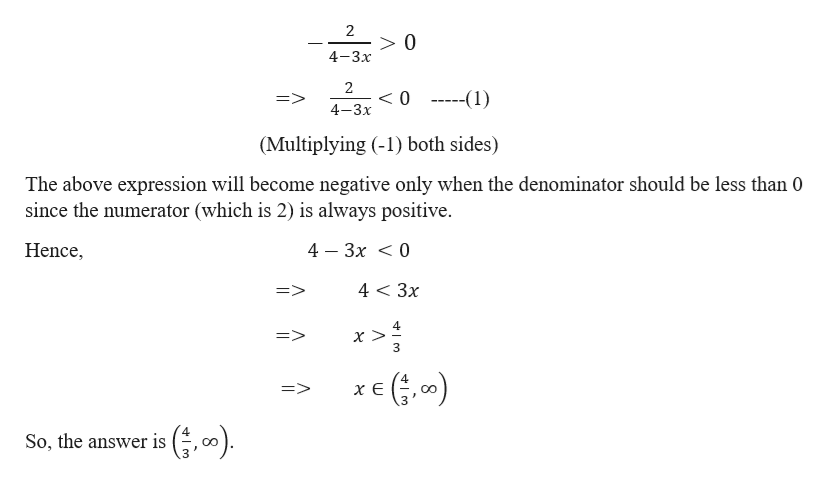# On a quiz I am given the equation to solve.-2/(4-3x)>0I am told to express the solution as an interval.The answer I gave was(-inf, 4/3)But I only received half credit for the answer.What did I do wrong, and what is the correct answer? Thanks.

Question
1 views

On a quiz I am given the equation to solve.

-2/(4-3x)>0

I am told to express the solution as an interval.

(-inf, 4/3)

What did I do wrong, and what is the correct answer? Thanks.

check_circle

star
star
star
star
star
1 Rating
Step 1

We need to find the interval of the given equation -2/(4-3x)>0.

Note:

First we will solve the given equation and then we will come back to the question that where you went wrong.

Step 2

So,

...help_outlineImage Transcriptionclose2 >0 4-3x 2 <0 ---(1) 4-3х (Multiplying (-1) both sides) The above expression will become negative only when the denominator should be less than 0 since the numerator (which is 2) is always positive. Hence, 4 — Зх < 0 4 < 3x => 4 => 3 => хе So, the answer is fullscreen

### Want to see the full answer?

See Solution

#### Want to see this answer and more?

Solutions are written by subject experts who are available 24/7. Questions are typically answered within 1 hour.*

See Solution
*Response times may vary by subject and question.
Tagged in

### Equations and In-equations